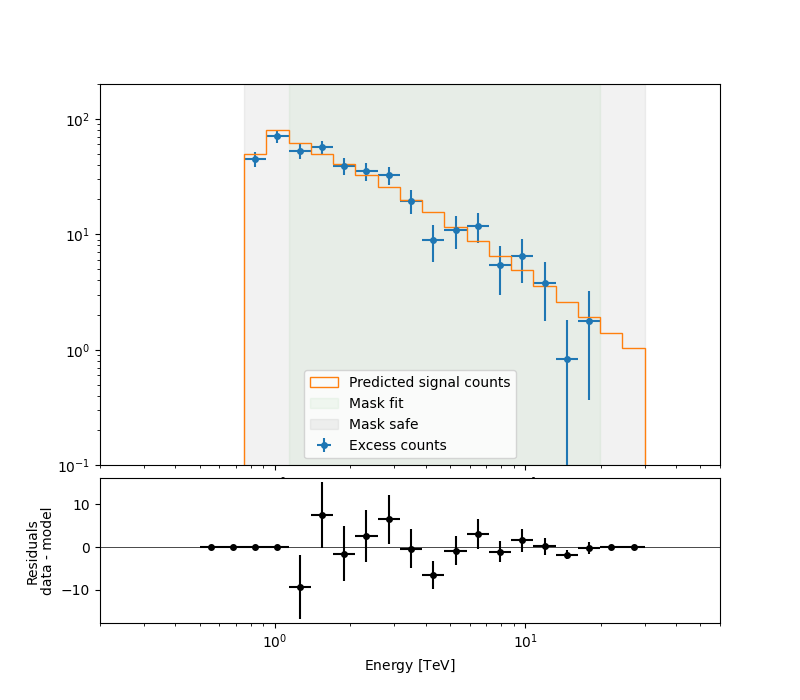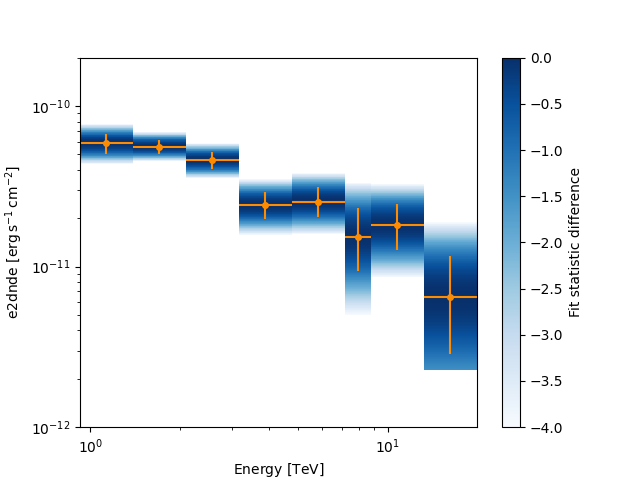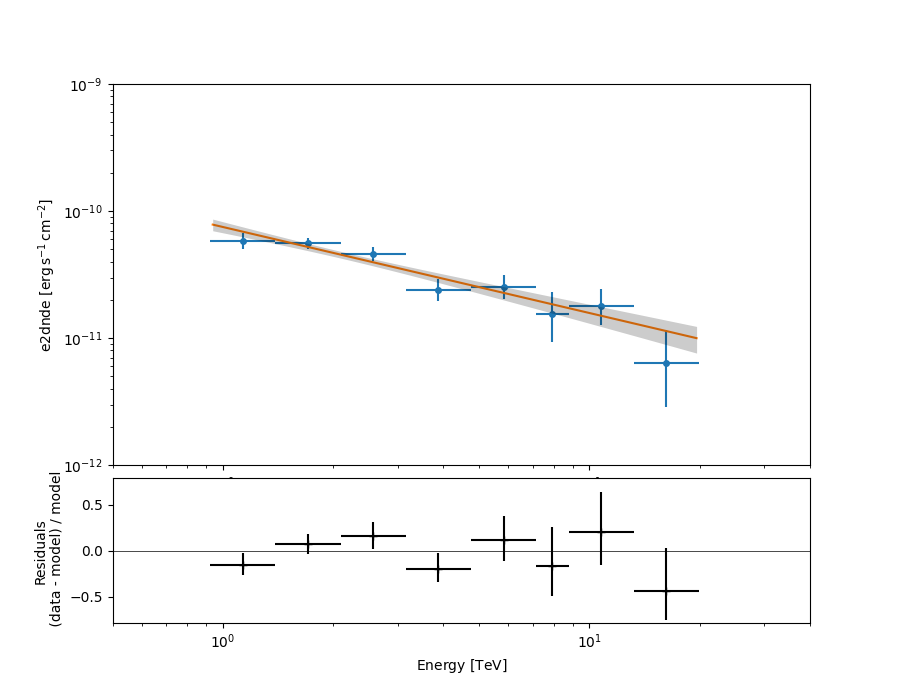# Spectral analysis with the HLI#

Introduction to 1D analysis using the Gammapy high level interface.

## Prerequisites#

• Understanding the gammapy data workflow, in particular what are DL3 events and instrument response functions (IRF).

## Context#

This notebook is an introduction to gammapy analysis using the high level interface.

Gammapy analysis consists in two main steps.

The first one is data reduction: user selected observations are reduced to a geometry defined by the user. It can be 1D (spectrum from a given extraction region) or 3D (with a sky projection and an energy axis). The resulting reduced data and instrument response functions (IRF) are called datasets in Gammapy.

The second step consists in setting a physical model on the datasets and fitting it to obtain relevant physical information.

Objective: Create a 1D dataset of the Crab using the H.E.S.S. DL3 data release 1 and perform a simple model fitting of the Crab nebula.

## Proposed approach#

This notebook uses the high level `Analysis` class to orchestrate data reduction and run the data fits. In its current state, `Analysis` supports the standard analysis cases of joint or stacked 3D and 1D analyses. It is instantiated with an `AnalysisConfig` object that gives access to analysis parameters either directly or via a YAML config file.

To see what is happening under-the-hood and to get an idea of the internal API, a second notebook performs the same analysis without using the `Analysis` class.

In summary, we have to:

• Create an `AnalysisConfig` object and the analysis configuration:

• Define what observations to use

• Define the geometry of the dataset (data and IRFs)

• Define the model we want to fit on the dataset.

• Instantiate a `Analysis` from this configuration and run the different analysis steps

• Observation selection

• Data reduction

• Model fitting

• Estimating flux points

```from pathlib import Path

# %matplotlib inline
import matplotlib.pyplot as plt
```

## Setup#

```from IPython.display import display
from gammapy.analysis import Analysis, AnalysisConfig
from gammapy.modeling.models import Models
```

## Check setup#

```from gammapy.utils.check import check_tutorials_setup

check_tutorials_setup()
```
```System:

python_executable      : /home/runner/work/gammapy-docs/gammapy-docs/gammapy/.tox/build_docs/bin/python
python_version         : 3.9.16
machine                : x86_64
system                 : Linux

Gammapy package:

version                : 1.1
path                   : /home/runner/work/gammapy-docs/gammapy-docs/gammapy/.tox/build_docs/lib/python3.9/site-packages/gammapy

Other packages:

numpy                  : 1.24.3
scipy                  : 1.10.1
astropy                : 5.2.2
regions                : 0.7
click                  : 8.1.3
yaml                   : 6.0
IPython                : 8.14.0
jupyterlab             : not installed
matplotlib             : 3.7.1
pandas                 : not installed
healpy                 : 1.16.2
iminuit                : 2.21.3
sherpa                 : 4.15.1
naima                  : 0.10.0
emcee                  : 3.1.4
corner                 : 2.2.2
ray                    : 2.5.0

Gammapy environment variables:

GAMMAPY_DATA           : /home/runner/work/gammapy-docs/gammapy-docs/gammapy-datasets/1.1
```

## Analysis configuration#

For configuration of the analysis we use the YAML data format. YAML is a machine-readable serialisation format, that is also friendly for humans to read. In this tutorial we will write the configuration file just using Python strings, but of course the file can be created and modified with any text editor of your choice.

Here is what the configuration for our analysis looks like:

```yaml_str = """
observations:
datastore: \$GAMMAPY_DATA/hess-dl3-dr1
obs_cone: {frame: icrs, lon: 83.633 deg, lat: 22.014 deg, radius: 5 deg}

datasets:
type: 1d
stack: true
geom:
axes:
energy: {min: 0.5 TeV, max: 30 TeV, nbins: 20}
energy_true: {min: 0.1 TeV, max: 50 TeV, nbins: 40}
on_region: {frame: icrs, lon: 83.633 deg, lat: 22.014 deg, radius: 0.11 deg}
containment_correction: true
methods: ['aeff-default', 'aeff-max']
parameters: {aeff_percent: 0.1}
background:
method: reflected
fit:
fit_range: {min: 1 TeV, max: 20 TeV}

flux_points:
energy: {min: 1 TeV, max: 20 TeV, nbins: 8}
source: 'crab'
"""

config = AnalysisConfig.from_yaml(yaml_str)
print(config)
```
```AnalysisConfig

general:
log: {level: info, filename: null, filemode: null, format: null, datefmt: null}
outdir: .
n_jobs: 1
datasets_file: null
models_file: null
observations:
datastore: \$GAMMAPY_DATA/hess-dl3-dr1
obs_ids: []
obs_file: null
obs_cone: {frame: icrs, lon: 83.633 deg, lat: 22.014 deg, radius: 5.0 deg}
obs_time: {start: null, stop: null}
required_irf: [aeff, edisp, psf, bkg]
datasets:
type: 1d
stack: true
geom:
wcs:
skydir: {frame: null, lon: null, lat: null}
binsize: 0.02 deg
width: {width: 5.0 deg, height: 5.0 deg}
binsize_irf: 0.2 deg
selection: {offset_max: 2.5 deg}
axes:
energy: {min: 0.5 TeV, max: 30.0 TeV, nbins: 20}
energy_true: {min: 0.1 TeV, max: 50.0 TeV, nbins: 40}
map_selection: [counts, exposure, background, psf, edisp]
background:
method: reflected
exclusion: null
parameters: {}
methods: [aeff-default, aeff-max]
parameters: {aeff_percent: 0.1}
on_region: {frame: icrs, lon: 83.633 deg, lat: 22.014 deg, radius: 0.11 deg}
containment_correction: true
fit:
fit_range: {min: 1.0 TeV, max: 20.0 TeV}
flux_points:
energy: {min: 1.0 TeV, max: 20.0 TeV, nbins: 8}
source: crab
parameters: {selection_optional: all}
excess_map:
parameters: {}
energy_edges: {min: null, max: null, nbins: null}
light_curve:
time_intervals: {start: null, stop: null}
energy_edges: {min: null, max: null, nbins: null}
source: source
parameters: {selection_optional: all}
```

Note that you can save this string into a yaml file and load it as follow:

```# config = AnalysisConfig.read("config-1d.yaml")
# # the AnalysisConfig gives access to the various parameters used from logging to reduced dataset geometries
# print(config)
```

### Using data stored into your computer#

Here, we want to use Crab runs from the H.E.S.S. DL3-DR1. We have defined the datastore and a cone search of observations pointing with 5 degrees of the Crab nebula. Parameters can be set directly or as a python dict.

PS: do not forget to set up your environment variable \$GAMMAPY_DATA to your local directory containing the H.E.S.S. DL3-DR1 as described in Quickstart Setup.

In order to properly adjust the background normalisation on regions without gamma-ray signal, one needs to define an exclusion mask for the background normalisation. For this tutorial, we use the following one `\$GAMMAPY_DATA/joint-crab/exclusion/exclusion_mask_crab.fits.gz`

```config.datasets.background.exclusion = (
)
```

We’re all set. But before we go on let’s see how to save or import `AnalysisConfig` objects though YAML files.

### Using YAML configuration files for setting/writing the Data Reduction parameters#

One can export/import the `AnalysisConfig` to/from a YAML file.

```config.write("config.yaml", overwrite=True)

print(config)
```
```AnalysisConfig

general:
log: {level: info, filename: null, filemode: null, format: null, datefmt: null}
outdir: .
n_jobs: 1
datasets_file: null
models_file: null
observations:
datastore: \$GAMMAPY_DATA/hess-dl3-dr1
obs_ids: []
obs_file: null
obs_cone: {frame: icrs, lon: 83.633 deg, lat: 22.014 deg, radius: 5.0 deg}
obs_time: {start: null, stop: null}
required_irf: [aeff, edisp, psf, bkg]
datasets:
type: 1d
stack: true
geom:
wcs:
skydir: {frame: null, lon: null, lat: null}
binsize: 0.02 deg
width: {width: 5.0 deg, height: 5.0 deg}
binsize_irf: 0.2 deg
selection: {offset_max: 2.5 deg}
axes:
energy: {min: 0.5 TeV, max: 30.0 TeV, nbins: 20}
energy_true: {min: 0.1 TeV, max: 50.0 TeV, nbins: 40}
map_selection: [counts, exposure, background, psf, edisp]
background:
method: reflected
parameters: {}
methods: [aeff-default, aeff-max]
parameters: {aeff_percent: 0.1}
on_region: {frame: icrs, lon: 83.633 deg, lat: 22.014 deg, radius: 0.11 deg}
containment_correction: true
fit:
fit_range: {min: 1.0 TeV, max: 20.0 TeV}
flux_points:
energy: {min: 1.0 TeV, max: 20.0 TeV, nbins: 8}
source: crab
parameters: {selection_optional: all}
excess_map:
parameters: {}
energy_edges: {min: null, max: null, nbins: null}
light_curve:
time_intervals: {start: null, stop: null}
energy_edges: {min: null, max: null, nbins: null}
source: source
parameters: {selection_optional: all}
```

## Running the first step of the analysis: the Data Reduction#

### Configuration of the analysis#

We first create an `Analysis` object from our configuration.

```analysis = Analysis(config)
```

### Observation selection#

We can directly select and load the observations from disk using `get_observations()`:

```analysis.get_observations()
```

The observations are now available on the `Analysis` object. The selection corresponds to the following ids:

```print(analysis.observations.ids)
```
```['23523', '23526', '23559', '23592']
```

To see how to explore observations, please refer to the following notebook: CTA with Gammapy or H.E.S.S. with Gammapy

### Running the Data Reduction#

Now we proceed to the data reduction. In the config file we have chosen a WCS map geometry, energy axis and decided to stack the maps. We can run the reduction using `get_datasets()`:

```analysis.get_datasets()
```

### Results exploration#

As we have chosen to stack the data, one can print what contains the unique entry of the datasets:

```print(analysis.datasets)
```
```SpectrumDatasetOnOff
--------------------

Name                            : stacked

Total counts                    : 427
Total background counts         : 25.86
Total excess counts             : 401.14

Predicted counts                : 43.14
Predicted background counts     : 43.14
Predicted excess counts         : nan

Exposure min                    : 2.90e+07 m2 s
Exposure max                    : 2.64e+09 m2 s

Number of total bins            : 20
Number of fit bins              : 18

Fit statistic type              : wstat
Fit statistic value (-2 log(L)) : 1396.10

Number of models                : 0
Number of parameters            : 0
Number of free parameters       : 0

Total counts_off                : 581
Acceptance                      : 20
Acceptance off                  : 495
```

As you can see the dataset uses WStat with the background computed with the Reflected Background method during the data reduction, but no source model has been set yet.

The counts, exposure and background, etc are directly available on the dataset and can be printed:

```info_table = analysis.datasets.info_table()
info_table

print(
f"Tobs={info_table['livetime'].to('h'):.1f} Excess={info_table['excess'].value:.1f} \
Significance={info_table['sqrt_ts']:.2f}"
)
```
```Tobs=1.8 h Excess=401.1 Significance=37.04
```

### Save dataset to disk#

It is common to run the preparation step independent of the likelihood fit, because often the preparation of counts, collection are and energy dispersion is slow if you have a lot of data. We first create a folder:

```path = Path("hli_spectrum_analysis")
path.mkdir(exist_ok=True)
```

And then write the stacked dataset to disk by calling the dedicated `write()` method:

```filename = path / "crab-stacked-dataset.fits.gz"
analysis.datasets.write(filename, overwrite=True)
```

## Model fitting#

### Creation of the model#

First, let’s create a model to be adjusted. As we are performing a 1D Analysis, only a spectral model is needed within the `SkyModel` object. Here is a pre-defined YAML configuration file created for this 1D analysis:

```model_str = """
components:
- name: crab
type: SkyModel
spectral:
type: PowerLawSpectralModel
parameters:
- name: index
frozen: false
scale: 1.0
unit: ''
value: 2.6
- name: amplitude
frozen: false
scale: 1.0
unit: cm-2 s-1 TeV-1
value: 5.0e-11
- name: reference
frozen: true
scale: 1.0
unit: TeV
value: 1.0
"""
model_1d = Models.from_yaml(model_str)
print(model_1d)
```
```Models

Component 0: SkyModel

Name                      : crab
Datasets names            : None
Spectral model type       : PowerLawSpectralModel
Spatial  model type       :
Temporal model type       :
Parameters:
index                         :      2.600   +/-    0.00
amplitude                     :   5.00e-11   +/- 0.0e+00 1 / (cm2 s TeV)
reference             (frozen):      1.000       TeV
```

Or from a yaml file, e.g.

```# model_1d = Models.read("model-1d.yaml")
# print(model_1d)
```

Now we set the model on the analysis object:

```analysis.set_models(model_1d)
```

### Setting fitting parameters#

`Analysis` can perform a few modeling and fitting tasks besides data reduction. Parameters have then to be passed to the configuration object.

### Running the fit#

```analysis.run_fit()
```

### Exploration of the fit results#

```print(analysis.fit_result)

display(model_1d.to_parameters_table())
```
```OptimizeResult

backend    : minuit
success    : True
message    : Optimization terminated successfully..
nfev       : 37
total stat : 10.29

CovarianceResult

backend    : minuit
method     : hesse
success    : True
message    : Hesse terminated successfully.

model   type      name     value         unit      ... max frozen is_norm link
----- -------- --------- ---------- -------------- ... --- ------ ------- ----
crab spectral     index 2.6768e+00                ... nan  False   False
crab spectral amplitude 4.6795e-11 cm-2 s-1 TeV-1 ... nan  False    True
crab spectral reference 1.0000e+00            TeV ... nan   True   False
```

To check the fit is correct, we compute the excess spectrum with the predicted counts.

```ax_spectrum, ax_residuals = analysis.datasets.plot_fit()
ax_spectrum.set_ylim(0.1, 200)
ax_spectrum.set_xlim(0.2, 60)
ax_residuals.set_xlim(0.2, 60)
plt.show()
```### Serialisation of the fit result#

This is how we can write the model back to file again:

```filename = path / "model-best-fit.yaml"
analysis.models.write(filename, overwrite=True)

with filename.open("r") as f:
```
```components:
-   name: crab
type: SkyModel
spectral:
type: PowerLawSpectralModel
parameters:
-   name: index
value: 2.676836990200946
error: 0.10350022010048233
-   name: amplitude
value: 4.679478012946556e-11
unit: cm-2 s-1 TeV-1
error: 4.678684168406788e-12
-   name: reference
value: 1.0
unit: TeV
covariance: model-best-fit_covariance.dat
```

## Creation of the Flux points#

### Running the estimation#

```analysis.get_flux_points()

crab_fp = analysis.flux_points.data
crab_fp_table = crab_fp.to_table(sed_type="dnde", formatted=True)
display(crab_fp_table)
```
```e_ref  e_min  e_max  ... success   norm_scan        stat_scan
TeV    TeV    TeV   ...
------ ------ ------ ... ------- -------------- ------------------
1.134  0.924  1.392 ...    True 0.200 .. 5.000  61.061 .. 323.310
1.708  1.392  2.096 ...    True 0.200 .. 5.000 137.686 .. 402.694
2.572  2.096  3.156 ...    True 0.200 .. 5.000 105.216 .. 245.371
3.873  3.156  4.753 ...    True 0.200 .. 5.000  30.617 .. 190.044
5.833  4.753  7.158 ...    True 0.200 .. 5.000   35.669 .. 89.296
7.929  7.158  8.784 ...    True 0.200 .. 5.000    5.680 .. 33.974
10.779  8.784 13.228 ...    True 0.200 .. 5.000   15.247 .. 34.090
16.233 13.228 19.921 ...    True 0.200 .. 5.000    2.084 .. 28.509
```

Let’s plot the flux points with their likelihood profile

```fig, ax_sed = plt.subplots()
crab_fp.plot(ax=ax_sed, sed_type="e2dnde", color="darkorange")
ax_sed.set_ylim(1.0e-12, 2.0e-10)
ax_sed.set_xlim(0.5, 40)
crab_fp.plot_ts_profiles(ax=ax_sed, sed_type="e2dnde")
plt.show()
```### Serialisation of the results#

The flux points can be exported to a fits table following the format defined here

```filename = path / "flux-points.fits"
analysis.flux_points.write(filename, overwrite=True)
```

## Plotting the final results of the 1D Analysis#

We can plot of the spectral fit with its error band overlaid with the flux points:

```ax_sed, ax_residuals = analysis.flux_points.plot_fit()
ax_sed.set_ylim(1.0e-12, 1.0e-9)
ax_sed.set_xlim(0.5, 40)
plt.show()
```## What’s next?#

You can look at the same analysis without the high level interface in Spectral analysis

As we can store the best model fit, you can overlay the fit results of both methods on a unique plot.

Total running time of the script: ( 0 minutes 10.828 seconds)

Gallery generated by Sphinx-Gallery